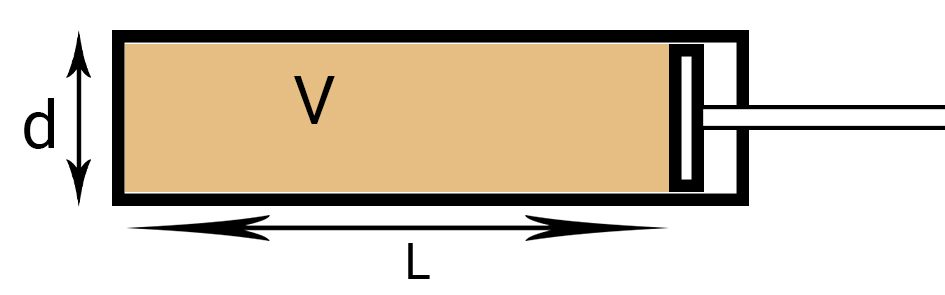# Engine Displacement Calculator

Created by Rahul Dhari
Reviewed by Steven Wooding
Last updated: Mar 08, 2022

The engine displacement calculator helps you determine the capacity or the cc value for an engine. This engine cc calculator will calculate the engine capacity using the dimensions of the cylinder and piston system. Ever wondered what does cc or cubic inch written alongside an engine means or how to find cc of an engine? Scroll down and read through our guide to understand better. Once you know the capacity of the engine, you can also calculate its compression ratio.

## What does cc mean in an engine – What is cc in an engine nomenclature?

You must have read about a 2 liter engine or a 100 cc motorcycle. The cc in an engine refers to the engine displacement in cubic centimeters. This capacity is estimated based on the volume swept by the piston, i.e., the volume of a cylinder. This parameter indicates the power generated by the engine and amount of fuel consumed. For example, a typical Formula One race car engine is of 1600 cc or 1.6 L capacity. The engine capacity is measured in cubic centimeters (cc) and cubic inches (cu. in.). For an engine with N cylinders, each having the bore diameter, D and depth, L, the engine displacement can be written as:

V = N * L * π * D2 / 4

The depth of the cylinder, L can also be written as stroke length. The stroke length is the distance traveled by the piston inside a cylinder. Interestingly, if you'll notice the term:

π * D2 * L / 4 = Volume

Therefore, engine displacement can also be referred to as:

Engine displacement = No. of cylinders * Volume of a single cylinder## How to use the engine displacement calculator?

Follow the steps below to know how to find engine displacement using our ingenious engine displacement calculator:

• Step 1: Count the number of cylinders for the engine, N.

• Step 2: Enter the bore diameter, D.

• Step 3: Input the stroke length, L.

• Step 4: The engine size calculator will return the engine displacement value in cubic centimeters (cc) or cubic inches (cu. in.).

## Example: Using the engine size calculator

Calculate the capacity of a 4 cylinder engine having bore diameter 50 mm and stroke length of 250 mm in cubic inches.

Given, no. of Cylinders, N = 4; bore diameter, D = 50 mm; and stroke length, L = 250 mm. Also, the problem statement requires the engine capacity in cubic inches. Let's set the unit for the engine displacement to cu in.

• Step 1: Identify the number of cylinders and input the appropriate value as N = 4.

• Step 2: Enter the bore diameter, D = 50 mm.

• Step 3: Use the stroke length, L = 250 mm.

• Step 4: The engine cubic inch calculator will now return the engine displacement value, such that:

V = 4 * 250 * π * 502 / 4 = 1,963,495 mm³ ≡ 119.82 cu. in.

Alternatively, you can calculate the diameter of the cylinder of stroke length using the engine displacement. Yes! The engine displacement calculator can work backward. For instance, let us determine the diameter of a cylinder for a 2-cylinder engine having a displacement of 200 cc with a stroke length of 150 mm.

Given, no. of cylinders, N = 2; stroke length, L = 150 mm; and engine displacement, V = 200 cc.

• Step 1: Enter the engine displacement, V = 200 cc.

• Step 2: Input the no. of cylinder as 2 and stroke length as 150 mm.

• Step 3: The engine cc calculator will provide you with the bore diameter which is 23.15 mm.

The calculation is performed as:

D = √((4 * V) / (N * π * L)) = √((4 * 200000) / (2 * π * 150)) = 23.15 mm

Rahul Dhari
Number of cylinders
Bore diameter
in
Stroke length
in
Engine displacement
cu in
People also viewed…

### Black Hole Collision Calculator

The Black Hole Collision Calculator lets you see the effects of a black hole collision, as well as revealing some of the mysteries of black holes, come on in and enjoy!

### Terminal velocity

Determine the maximum velocity achievable by a falling object using the terminal velocity calculator.

### Titration

Use our titration calculator to determine the molarity of your solution.

### Wire resistance

Wire resistance calculator determines the resistance and the conductance of a wire.